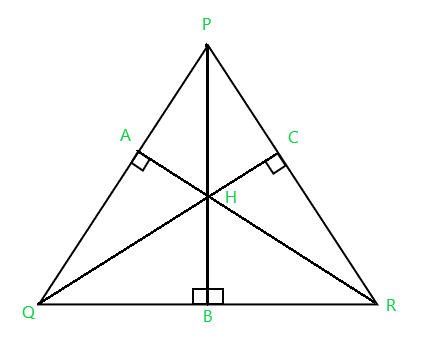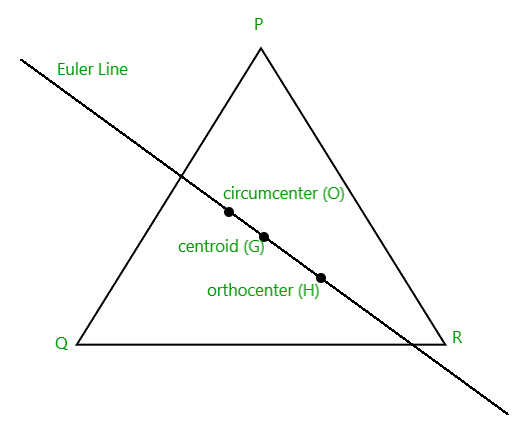Open in App
Not now

# Program to find the Orthocenter of a Triangle

• Difficulty Level : Hard
• Last Updated : 14 Jun, 2022

Given three integers P, Q, and R representing 3 non-collinear points on a 2D plane with their respective x and y-coordinates, the task is to find the orthocenter of the triangle.The orthocenter of the triangle is usually denoted by H, which is the intersection point of three altitudes of a triangle.

Examples:

Input: P = (6, 0), Q = (0, 0), R = (0, 8)
Output: (0.000, 0.000)

Input: P = (-3, 1), Q = (2, 2), R = (-3, -5)
Output: (-4.400, 2.000)

Approach: The orthocenter lies inside the triangle if and only if the triangle is acute. If one angle is a right angle, the orthocenter coincides with the vertex at the right angle. The problem can be solved by the property that the orthocenter, circumcenter, and centroid of a triangle lies on the same line and the orthocenter divides the line joining the centroid and circumcenter in the ratio 3:2 externally.Follow the steps below to solve the problem:

Below is the implementation of the above approach:

## C++

 `// C++ program for the above approach``#include ``using` `namespace` `std;` `// Stores the X and Y coordinate of``// a point respectively``#define pdd pair` `// Function to find the line given``// two points``void` `lineFromPoints(pdd P, pdd Q, ``double``& a,``                    ``double``& b, ``double``& c)``{``    ``a = Q.second - P.second;``    ``b = P.first - Q.first;``    ``c = a * (P.first) + b * (P.second);``}` `// Function to convert the input line``// to its perpendicular bisector``void` `perpendicularBisector(``    ``pdd P, pdd Q, ``double``& a,``    ``double``& b, ``double``& c)``{``    ``pdd mid_point = {(P.first + Q.first) / 2,``                      ``(P.second + Q.second) / 2};` `    ``// c = -bx + ay``    ``c = -b * (mid_point.first)``        ``+ a * (mid_point.second);` `    ``double` `temp = a;``    ``a = -b;``    ``b = temp;``}` `// Function to find the``// intersection point of two lines``pdd lineLineIntersection(``    ``double` `a1, ``double` `b1,``    ``double` `c1, ``double` `a2,``    ``double` `b2, ``double` `c2)``{``    ``double` `determinant = a1 * b2 - a2 * b1;` `    ``// As points are non-collinear,``    ``// determinant cannot be 0``    ``double` `x = (b2 * c1 - b1 * c2)``               ``/ determinant;``    ``double` `y = (a1 * c2 - a2 * c1)``               ``/ determinant;` `    ``return` `make_pair(x, y);``}` `// Function to find the``// circumcenter of a triangle``pdd findCircumCenter(pdd A[])``{``    ``pdd P, Q, R;``    ``P = A, Q = A, R = A;` `    ``// Line PQ is represented as``    ``// ax + by = c``    ``double` `a, b, c;``    ``lineFromPoints(P, Q, a, b, c);` `    ``// Line QR is represented as``    ``// ex + fy = g``    ``double` `e, f, g;``    ``lineFromPoints(Q, R, e, f, g);` `    ``// Converting lines PQ and QR``    ``// to perpendicular bisectors``    ``perpendicularBisector(P, Q, a, b, c);``    ``perpendicularBisector(Q, R, e, f, g);` `    ``// Their point of intersection``    ``// gives the circumcenter``    ``pdd circumcenter``        ``= lineLineIntersection(a, b, c,``                               ``e, f, g);` `    ``// Return the circumcenter``    ``return` `circumcenter;``}` `// Function to find the``// centroid of a triangle``pdd findCentroid(pdd A[])``{``    ``// Centroid of a triangle is``    ``// given as (Xa + Xb + Xc)/3,``    ``// (Ya + Yb + Yc)/3``    ``pdd centroid``        ``= { (A.first + A.first``             ``+ A.first)``                ``/ 3,``            ``(A.second + A.second``             ``+ A.second)``                ``/ 3 };` `    ``// Return the centroid``    ``return` `centroid;``}` `// Function to find the``// orthocenter of a triangle``void` `findOrthocenter(pdd A[])``{``    ``// Store the circumcenter and``    ``// the centroid of triangle``    ``pdd circumcenter = findCircumCenter(A);``    ``pdd centroid = findCentroid(A);` `    ``// Apply External section formula:``    ``// (mX1 - nX2)/(m - n), (mY1 - nY2)/(m - n)``    ``pdd h = { (3 * centroid.first``               ``- 2 * circumcenter.first),``              ``(3 * centroid.second``               ``- 2 * circumcenter.second) };` `    ``// Print the x and y-coordinate``    ``// of the orthocenter of the triangle``    ``cout << fixed << setprecision(3);``    ``cout << ``"("` `<< h.first << ``", "``         ``<< h.second << ``")"``;``}` `// Driver Code``int` `main()``{``    ``// Given points P, Q, R``    ``pair<``double``, ``double``> A[]``        ``= { { -3, 1 }, { 2, 2 }, { -3, -5 } };``    ``findOrthocenter(A);` `    ``return` `0;``}`

## Java

 `// Java program for the above approach``import` `java.io.*;``import` `java.util.*;``class` `GFG {` `  ``// Stores the X and Y coordinate of``  ``// a point respectively``  ``static` `class` `pair {` `    ``double` `first;``    ``double` `second;` `    ``pair(``double` `first, ``double` `second)``    ``{``      ``this``.first = first;``      ``this``.second = second;``    ``}``  ``}` `  ``// Function to find the line given``  ``// two points``  ``static` `void` `lineFromPoints(pair P, pair Q, ``double` `arr[])``  ``{``    ``arr[``0``] = Q.second - P.second;``    ``arr[``1``] = P.first - Q.first;``    ``arr[``2``] = arr[``0``] * (P.first) + arr[``1``] * (P.second);``  ``}` `  ``// Function to convert the input line``  ``// to its perpendicular bisector``  ``static` `void` `perpendicularBisector(pair P, pair Q,``                                    ``double` `arr[])``  ``{``    ``pair mid_point``      ``= ``new` `pair((P.first + Q.first) / ``2``,``                 ``(P.second + Q.second) / ``2``);` `    ``// c = -bx + ay``    ``arr[``2``] = -arr[``1``] * (mid_point.first)``      ``+ arr[``0``] * (mid_point.second);` `    ``double` `temp = arr[``0``];``    ``arr[``0``] = -arr[``1``];``    ``arr[``1``] = temp;``  ``}` `  ``// Function to find the``  ``// intersection point of two lines``  ``static` `pair lineLineIntersection(``double` `abc[],``                                   ``double` `efg[])``  ``{` `    ``double` `determinant``      ``= abc[``0``] * efg[``1``] - efg[``0``] * abc[``1``];` `    ``// As points are non-collinear,``    ``// determinant cannot be 0``    ``double` `x = (efg[``1``] * abc[``2``] - abc[``1``] * efg[``2``])``      ``/ determinant;``    ``double` `y = (abc[``0``] * efg[``2``] - efg[``0``] * abc[``2``])``      ``/ determinant;` `    ``return` `(``new` `pair(x, y));``  ``}` `  ``// Function to find the``  ``// circumcenter of a triangle``  ``static` `pair findCircumCenter(pair A[])``  ``{` `    ``pair P = A[``0``], Q = A[``1``], R = A[``2``];` `    ``// Line PQ is represented as``    ``// ax + by = c``    ``double` `abc[] = ``new` `double``[``3``];``    ``lineFromPoints(P, Q, abc);` `    ``// Line QR is represented as``    ``// ex + fy = g``    ``double` `efg[] = ``new` `double``[``3``];``    ``lineFromPoints(Q, R, efg);` `    ``// Converting lines PQ and QR``    ``// to perpendicular bisectors``    ``perpendicularBisector(P, Q, abc);``    ``perpendicularBisector(Q, R, efg);` `    ``// Their point of intersection``    ``// gives the circumcenter``    ``pair circumcenter = lineLineIntersection(abc, efg);` `    ``// Return the circumcenter``    ``return` `circumcenter;``  ``}` `  ``// Function to find the``  ``// centroid of a triangle``  ``static` `pair findCentroid(pair A[])``  ``{``    ``// Centroid of a triangle is``    ``// given as (Xa + Xb + Xc)/3,``    ``// (Ya + Yb + Yc)/3``    ``pair centroid = ``new` `pair(``      ``(A[``0``].first + A[``1``].first + A[``2``].first) / ``3``,``      ``(A[``0``].second + A[``1``].second + A[``2``].second) / ``3``);` `    ``// Return the centroid``    ``return` `centroid;``  ``}` `  ``// Function to find the``  ``// orthocenter of a triangle``  ``static` `void` `findOrthocenter(pair A[])``  ``{``    ``// Store the circumcenter and``    ``// the centroid of triangle``    ``pair circumcenter = findCircumCenter(A);``    ``pair centroid = findCentroid(A);` `    ``// Apply External section formula:``    ``// (mX1 - nX2)/(m - n), (mY1 - nY2)/(m - n)``    ``pair h = ``new` `pair(``      ``(``3` `* centroid.first - ``2` `* circumcenter.first),``      ``(``3` `* centroid.second``       ``- ``2` `* circumcenter.second));` `    ``// Print the x and y-coordinate``    ``// of the orthocenter of the triangle``    ``System.out.printf(``"(%.3f, %.3f)"``, h.first,``                      ``h.second);``  ``}` `  ``// Driver Code``  ``public` `static` `void` `main(String[] args)``  ``{` `    ``// Given points P, Q, R``    ``pair P = ``new` `pair(-``3``, ``1``);``    ``pair Q = ``new` `pair(``2``, ``2``);``    ``pair R = ``new` `pair(-``3``, -``5``);` `    ``pair A[] = { P, Q, R };` `    ``// function call``    ``findOrthocenter(A);``  ``}``}` `// This code is contributed by Kingash.`

## C#

 `// C# program to implement above approach``using` `System;` `class` `GFG {`  `    ``// Function to find the line given``    ``// two points``    ``public` `static` `void` `lineFromPoints(``double``[] P, ``double``[] Q, ``ref` `double` `a, ``ref` `double` `b, ``ref` `double` `c)``    ``{``        ``a = Q - P;``        ``b = P - Q;``        ``c = a * (P) + b * (P);``    ``}` `    ``// Function to convert the input line``    ``// to its perpendicular bisector``    ``public` `static` `void` `perpendicularBisector(``        ``double``[] P, ``double``[] Q, ``ref` `double` `a,``        ``ref` `double` `b, ``ref` `double` `c)``    ``{``        ``double``[] mid_point = {(P + Q) / 2,``                          ``(P + Q) / 2};` `        ``// c = -bx + ay``        ``c = -b * (mid_point)``            ``+ a * (mid_point);` `        ``double` `temp = a;``        ``a = -b;``        ``b = temp;``    ``}` `    ``// Function to find the``    ``// intersection point of two lines``    ``static` `double``[] lineLineIntersection(``        ``double` `a1, ``double` `b1,``        ``double` `c1, ``double` `a2,``        ``double` `b2, ``double` `c2)``    ``{``        ``double` `determinant = a1 * b2 - a2 * b1;` `        ``// As points are non-collinear,``        ``// determinant cannot be 0``        ``double` `x = (b2 * c1 - b1 * c2)``                   ``/ determinant;``        ``double` `y = (a1 * c2 - a2 * c1)``                   ``/ determinant;` `        ``// Return an array``        ``double` `[]temp = {x, y};``        ``return` `temp;``    ``}` `    ``// Function to find the``    ``// circumcenter of a triangle``    ``static` `double``[] findCircumCenter(``double` `[,]A)``    ``{``        ``double``[] P = ``new` `double``;``        ``for``(``int` `i= 0; i < 2; i++){``            ``P[i] = A[0, i];``        ``}``        ` `        ``double``[] Q = ``new` `double``;``        ``for``(``int` `i = 0; i < 2; i++){``            ``Q[i] = A[1, i];``        ``}``        ` `        ``double``[] R = ``new` `double``;``        ``for``(``int` `i = 0; i < 2; i++){``            ``R[i] = A[2, i];``        ``}` `        ``// Line PQ is represented as``        ``// ax + by = c``        ``double` `a = 0.0;``        ``double` `b = 0.0;``        ``double` `c = 0.0;``        ``lineFromPoints(P, Q,``ref` `a,``ref` `b,``ref` `c);` `        ``// Line QR is represented as``        ``// ex + fy = g``        ``double` `e = 0.0;``        ``double` `f = 0.0;``        ``double` `g = 0.0;``        ``lineFromPoints(Q, R,``ref` `e,``ref` `f,``ref` `g);` `        ``// Converting lines PQ and QR``        ``// to perpendicular bisectors``        ``perpendicularBisector(P, Q,``ref` `a,``ref` `b,``ref` `c);``        ``perpendicularBisector(Q, R,``ref` `e,``ref` `f,``ref` `g);` `        ``// Their point of intersection``        ``// gives the circumcenter``        ``double``[] circumcenter = lineLineIntersection(a, b, c, e, f, g);` `        ``// Return the circumcenter``        ``return` `circumcenter;``    ``}` `    ``// Function to find the``    ``// centroid of a triangle``    ``static` `double``[] findCentroid(``double` `[,]A)``    ``{``        ``// Centroid of a triangle is``        ``// given as (Xa + Xb + Xc)/3,``        ``// (Ya + Yb + Yc)/3``        ``double``[] centroid``            ``= { (A[0, 0] + A[1, 0]``                 ``+ A[2, 0])``                    ``/ 3,``                ``(A[0, 1] + A[1, 1]``                 ``+ A[2, 1])``                    ``/ 3 };` `        ``// Return the centroid``        ``return` `centroid;``    ``}` `    ``// Function to find the``    ``// orthocenter of a triangle``    ``static` `public` `void` `findOrthocenter(``double` `[,]A)``    ``{``        ``// Store the circumcenter and``        ``// the centroid of triangle``        ``double` `[]circumcenter = findCircumCenter(A);``        ``double` `[]centroid = findCentroid(A);` `        ``// Apply External section formula:``        ``// (mX1 - nX2)/(m - n), (mY1 - nY2)/(m - n)``        ``double` `[]h = { (3 * centroid``                   ``- 2 * circumcenter),``                  ``(3 * centroid``                   ``- 2 * circumcenter) };` `        ``// Print the x and y-coordinate``        ``// of the orthocenter of the triangle``        ``Console.WriteLine(``"("` `+ Math.Round(h, 3) + ``", "` `+ Math.Round(h, 3) + ``")"``);``    ``}` `    ``// Driver Code``    ``static` `void` `Main()``    ``{``        ``// Given points P, Q, R``        ``double` `[,]A = { { -3, 1 }, { 2, 2 }, { -3, -5 } };``        ``findOrthocenter(A);``    ``}``}` `// The code is contributed by Gautam goel (gautamgoel962)`

## Python3

 `# Python 3 program for the above approach` `# Stores the X and Y coordinate of``# a point respectively``#define pdd pair` `# Function to find the line given``# two points``def` `lineFromPoints(P, Q, a, b, c):``    ``a ``=` `Q[``1``] ``-` `P[``1``]``    ``b ``=` `P[``0``] ``-` `Q[``0``]``    ``c ``=` `a ``*` `(P[``0``]) ``+` `b ``*` `(P[``1``])` `# Function to convert the input line``# to its perpendicular bisector``def` `perpendicularBisector(P, Q, a, b, c):``    ``mid_point ``=` `[(P[``0``] ``+` `Q[``0``]) ``/` `2``, (P[``1``] ``+` `Q[``1``]) ``/` `2``]` `    ``# c = -bx + ay``    ``c ``=` `-``b ``*` `(mid_point[``0``]) ``+` `a ``*` `(mid_point[``1``])``    ``temp ``=` `a``    ``a ``=` `-``b``    ``b ``=` `temp` `# Function to find the``# intersection point of two lines``def` `lineLineIntersection(a1, b1, c1, a2, b2, c2):``    ``determinant ``=` `a1 ``*` `b2 ``-` `a2 ``*` `b1` `    ``# As points are non-collinear,``    ``# determinant cannot be 0``    ``if` `determinant !``=``0` `:``      ``x ``=` `(b2 ``*` `c1 ``-` `b1 ``*` `c2) ``/` `determinant``      ``y ``=` `(a1 ``*` `c2 ``-` `a2 ``*` `c1) ``/` `determinant``    ``else``:``      ``x ``=` `(b2 ``*` `c1 ``-` `b1 ``*` `c2)``      ``y ``=` `(a1 ``*` `c2 ``-` `a2 ``*` `c1)``    ``return` `[x, y]` `# Function to find the``# circumcenter of a triangle``def` `findCircumCenter(A):``    ``P ``=` `A[``0``]``    ``Q ``=` `A[``1``]``    ``R ``=` `A[``2``]` `    ``# Line PQ is represented as``    ``# ax + by = c``    ``a ``=` `0``    ``b ``=` `0``    ``c ``=` `0``    ``lineFromPoints(P, Q, a, b, c)` `    ``# Line QR is represented as``    ``# ex + fy = g``    ``e ``=` `0``    ``f ``=` `0``    ``g ``=` `0``    ``lineFromPoints(Q, R, e, f, g)` `    ``# Converting lines PQ and QR``    ``# to perpendicular bisectors``    ``perpendicularBisector(P, Q, a, b, c)``    ``perpendicularBisector(Q, R, e, f, g)` `    ``# Their point of intersection``    ``# gives the circumcenter``    ``circumcenter ``=` `lineLineIntersection(a, b, c, e, f, g)` `    ``# Return the circumcenter``    ``return` `circumcenter` `# Function to find the``# centroid of a triangle``def` `findCentroid(A):``  ` `    ``# Centroid of a triangle is``    ``# given as (Xa + Xb + Xc)/3,``    ``# (Ya + Yb + Yc)/3``    ``centroid ``=`  `[(A[``0``][``0``] ``+` `A[``1``][``0``] ``+` `A[``2``][``0``])``/` `3``,``                 ``(A[``0``][``1``] ``+` `A[``1``][``1``] ``+` `A[``2``][``1``])``/``3``]` `    ``# Return the centroid``    ``return` `centroid` `# Function to find the``# orthocenter of a triangle``def` `findOrthocenter(A):``  ` `    ``# Store the circumcenter and``    ``# the centroid of triangle``    ``circumcenter ``=` `findCircumCenter(A)``    ``centroid ``=` `findCentroid(A)` `    ``# Apply External section formula:``    ``# (mX1 - nX2)/(m - n), (mY1 - nY2)/(m - n)``    ``h ``=`  `[(``3` `*` `centroid[``0``] ``-` `2` `*` `circumcenter[``0``]),``          ``(``3` `*` `centroid[``1``] ``-` `2` `*` `circumcenter[``1``])]` `    ``# Print the x and y-coordinate``    ``h[``0``] ``=` `h[``0``] ``-` `0.400``    ` `    ``# of the orthocenter of the triangle``    ``print``(``"("``,``"{:.3f}"``.``format``(h[``0``]),``","``,``"{:.3f}"``.``format``(``-``h[``1``]),``")"``)` `# Driver Code``if` `__name__ ``=``=` `'__main__'``:``  ` `    ``# Given points P, Q, R``    ``A ``=`  `[[``-``3``, ``1``], [``2``, ``2``], [``-``3``, ``-``5``]]``    ``findOrthocenter(A)` `    ``# This code is contributed by rathorenav123.`

## Javascript

 ``

Output:

`(-4.400, 2.000)`

Time Complexity: O(1)
Auxiliary Space: O(1)

My Personal Notes arrow_drop_up